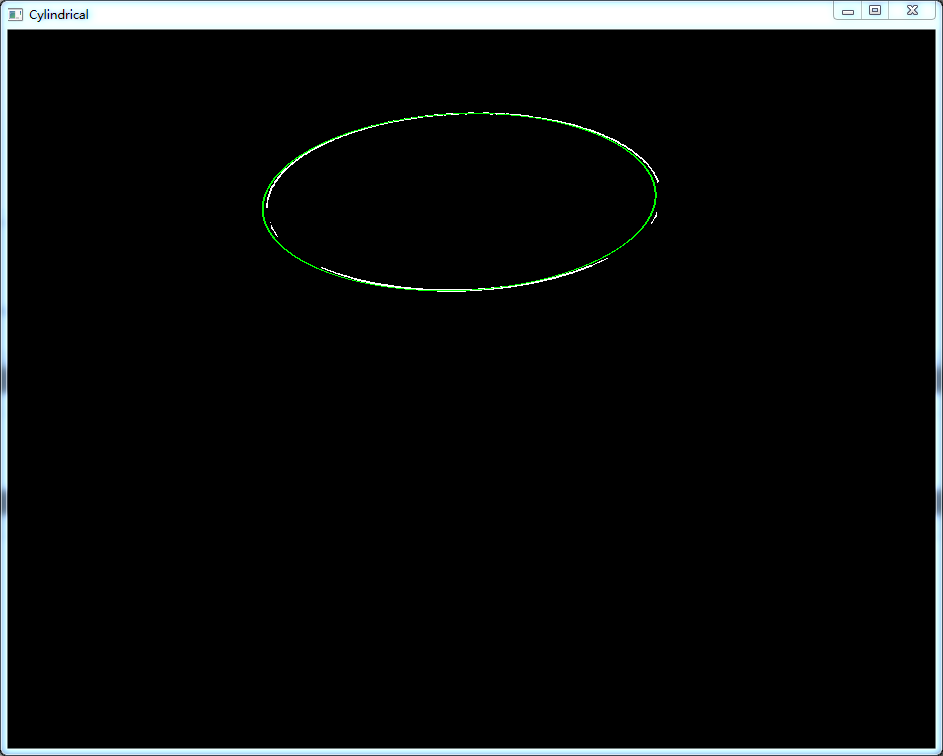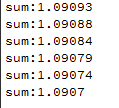2017-09-27 10:59:08 xiao_lxl 阅读数 2248
• ###### Qt项目实战之网络电子白板

本课程使用Qt技术实现了网络电子白板，支持直线、矩形、椭圆、三角形、涂鸦等图形元素。本课程实现的电子白板，可以在多人之间共享，每个人都可以进行任意绘制，每个人的绘制都可以同步显示在其它人的白板上。服务器端使用Qt Network开发，客户端使用Qt Network和Qt Graphics View Framework开发，数据传输使用JSON数据格式。

11506 人正在学习 去看看 安晓辉

《皮肤检测技术的研究及改进》``````    void cvSkinSegment(IplImage* img, IplImage* mask)   //原始图像及目标图像
{
CvSize imageSize = cvSize(img->width, img->height);
IplImage *imgY = cvCreateImage(imageSize, IPL_DEPTH_8U, 1);
IplImage *imgCr = cvCreateImage(imageSize, IPL_DEPTH_8U, 1);
IplImage *imgCb = cvCreateImage(imageSize, IPL_DEPTH_8U, 1);

IplImage *imgYCrCb = cvCreateImage(imageSize, img->depth, img->nChannels);
cvCvtColor(img,imgYCrCb,CV_BGR2YCrCb);
cvSplit(imgYCrCb, imgY, imgCr, imgCb, 0);   //得到每一通道的数据
int y, cr, cb, l, x1, y1, value;
unsigned char *pY, *pCr, *pCb, *pMask;

pY = (unsigned char *)imgY->imageData;  //Y通道的第一行数据的指针
pCr = (unsigned char *)imgCr->imageData;
pCb = (unsigned char *)imgCb->imageData;
l = img->height * img->width;   //图像的元素个数
for (int i = 0; i < l; i++)
{
y  = *pY;
cr = *pCr;
cb = *pCb;
cb -= 109;
cr -= 152;
x1 = (819*cr-614*cb)/32 + 51;
y1 = (819*cr+614*cb)/32 + 77;
x1 = x1*41/1024;
y1 = y1*73/1024;
value = x1*x1+y1*y1;    //构造椭圆的模型
if(y<100)    (*pMask)=(value<700) ? 255:0;    //对齐进行值得选择，要不255，要不0  //在不同的亮度下呈现出不同的亮度
else        (*pMask)=(value<850)? 255:0;    //255 is the skin, 0 is the background   //255 纯白色 0 纯黑色
pY++;
pCr++;
pCb++;
}

cvReleaseImage(&imgY);
cvReleaseImage(&imgCr);
cvReleaseImage(&imgCb);
cvReleaseImage(&imgYCrCb);
}
``````

2019-05-16 10:11:18 cheng_xing_ 阅读数 804
• ###### Qt项目实战之网络电子白板

本课程使用Qt技术实现了网络电子白板，支持直线、矩形、椭圆、三角形、涂鸦等图形元素。本课程实现的电子白板，可以在多人之间共享，每个人都可以进行任意绘制，每个人的绘制都可以同步显示在其它人的白板上。服务器端使用Qt Network开发，客户端使用Qt Network和Qt Graphics View Framework开发，数据传输使用JSON数据格式。

11506 人正在学习 去看看 安晓辉

# 经典椭圆检测方法

## 投票（聚类）方法

### 随机hough变换椭圆检测算法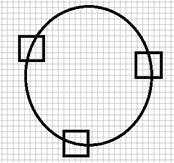1.把边缘检测得到的点收进集合V中，失败计数器f初始值设为0。设定5个阈值，分别是能容忍的失败次数最大值Tf，检测进行时对V中边缘点数量的要求阈值Tem，随机选取的三点之间两两距离最小值Ta，随机选取的第四点到可能椭圆边界距离的最大值Td，以及椭圆残缺比率阈值Tr。
2.np表示集合V中剩余的点的数量，当np小于Tem时或当失败次数f大于Tf时停止检测，算法终止；否则从V中随机取四点，并从V中删除这四点。
3.若用来求解椭圆参数的三个点两两之间距离都大于Ta，拟合出椭圆，计算第四个点到该椭圆边界的距离，若距离小于Td，执行第4步；若不满足两者之一，将这四个点返回到V中，失败次数加一，回到第2步执行。
4.设E为第3步拟合出来的椭圆，初始化满足阈值Td的点的个数num。遍历V中点，计算并判断它们到椭圆E的边界的距离是否小于Td，若是则num=num+1，并将该点从V中除去，直到遍历完成。
5.若num>=Tr*K，其中K为椭圆E的周长，那么跳转到第6步；否则认为椭圆E不是真实的椭圆，将第4步和第2步中删除的num+4个点返回V中，并跳转到第2步。
6.认为椭圆E是一个真实存在的椭圆，f置0，并跳转到第2步。

# 边界聚类算法流程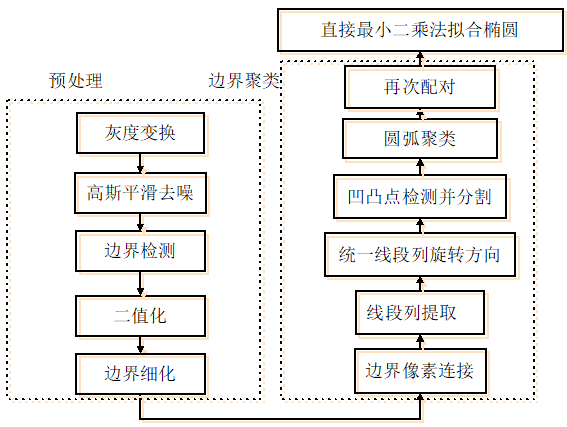## 预处理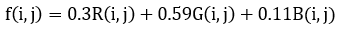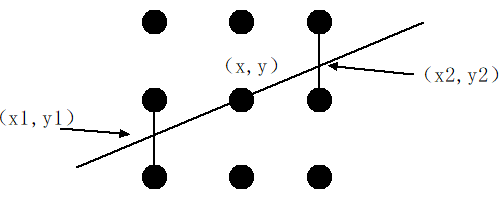## 边界像素连接

1.以8-邻域连通准则从上至下，从左至右扫描二值图像，按连通区域对图像中的像素点聚类。
2.寻找每一个连通域中边界像素中所有的结束点和分叉点（分叉点是三条以上曲线的交点）并存储。
3.以这些结束点和分叉点为结束标志，让每一个连通域中的像素点集合分割为遇到结束点和连接点就断开的小集合。
4.删除这些集合中像素数小于某一阈值的部分。

## 线段列提取

1.取边界像素连接成的第i条有向边界列，判断是否超过边界列总数目total，若不是进行步骤2，若是终止算法。
2.判断该边界列是否已经完成处理，若为否则进行第3步；若是则i=i+1，重新进行步骤2.
3.从其中第三个点开始，计算第一个点到这个点(记为点j)的连线方程，并依次判断第一个点和该点之间的所有点到该连线的距离，若所有距离均小于某一阈值，则j=j+1，重新进行步骤3，否则该有向边界列从该处断开，前面部分只保留第一个点和第j个点，前面j个点构成的连线用第一点和第j点之间的直线连线代替；后面部分仍然记为边界列i，
4.判断步骤3中断开的有向边界列后面部分像素数是否小于某阈值，若是则删除掉，否则不处理。最后跳转到步骤1。

## 线段列旋转方向统一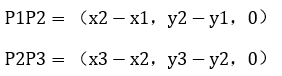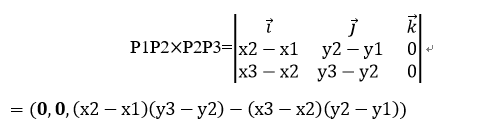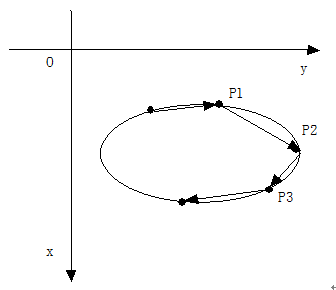## 凹点和角点检测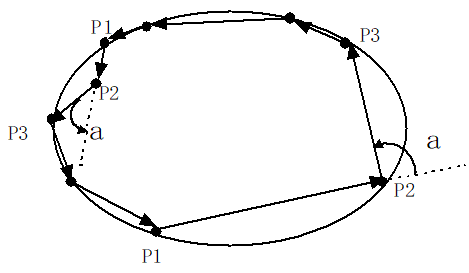## 圆弧聚类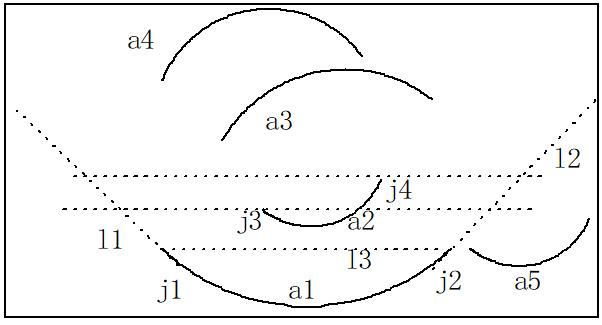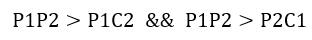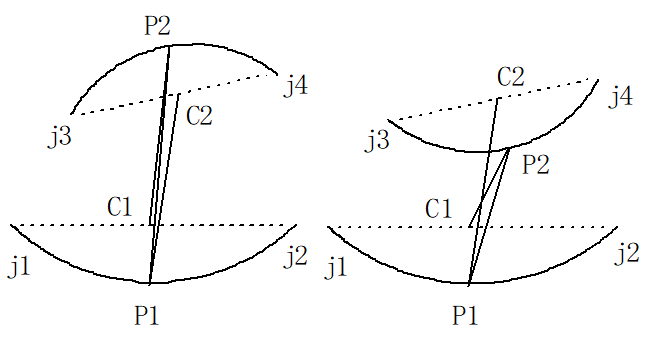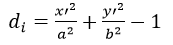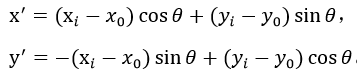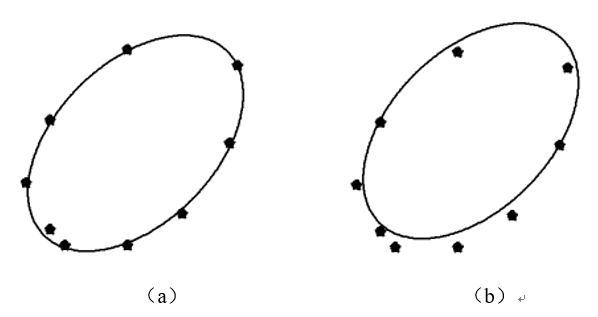# 实验效果

## 边界聚类算法检测结果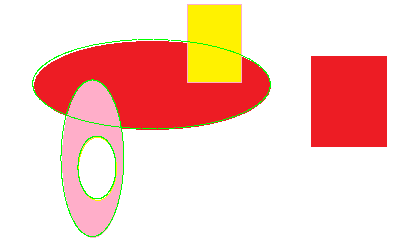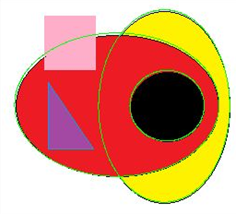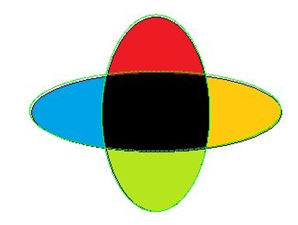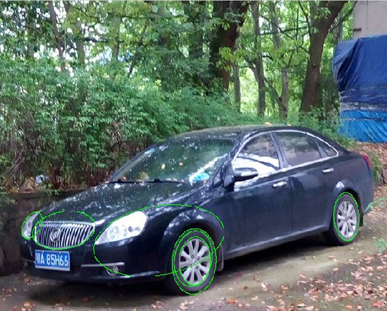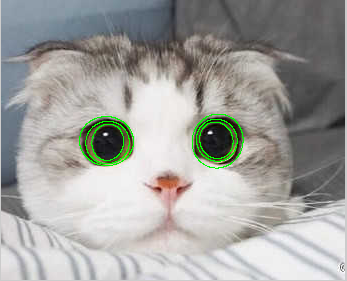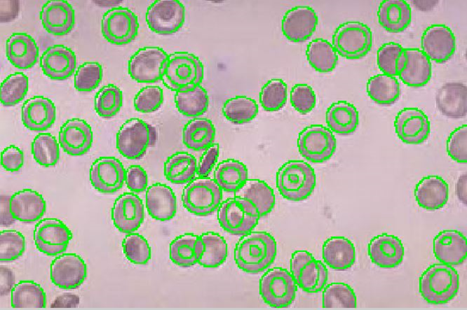## 边界聚类算法和随机霍夫变换算法比较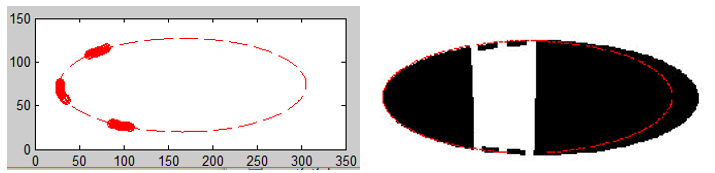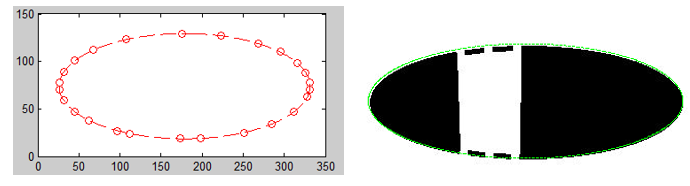2019-03-16 14:32:13 weixin_44540503 阅读数 945
• ###### Qt项目实战之网络电子白板

本课程使用Qt技术实现了网络电子白板，支持直线、矩形、椭圆、三角形、涂鸦等图形元素。本课程实现的电子白板，可以在多人之间共享，每个人都可以进行任意绘制，每个人的绘制都可以同步显示在其它人的白板上。服务器端使用Qt Network开发，客户端使用Qt Network和Qt Graphics View Framework开发，数据传输使用JSON数据格式。

11506 人正在学习 去看看 安晓辉

## 利用opencv进行图像处理，提取椭圆圆心处理

1，二值化处理（threhold()）
2，高斯滤波（GaussianBlur()）
3,轮廓提取（canny算子）
4，寻找闭合轮廓（findContours()）

1，像素点数量过滤（size）
2，面积过滤
3，长宽比过滤

``````#include<iostream>
#include"opencv.hpp"

using namespace std;
using namespace cv;

void drawCross(Mat &img, Point2f point, Scalar color, int size, int thickness /*= 1*/)
{
//绘制横线
line(img, cvPoint(point.x - size / 2, point.y), cvPoint(point.x + size / 2, point.y), color, thickness, 8, 0);
//绘制竖线
line(img, cvPoint(point.x, point.y - size / 2), cvPoint(point.x, point.y + size / 2), color, thickness, 8, 0);
return;
}

//提取单幅图像的特征点
void FeaturePoint(Mat &img)
{
Point2f center; //定义变量
vector<Point2f> ellipsecenterleft;
Mat edges;
threshold(img, img, 80, 255, CV_THRESH_BINARY_INV);
//imshow("threshold", img);
////此函数等待按键，按键盘任意键就返回
//waitKey(0);
GaussianBlur(img, edges, Size(5, 5), 0, 0);
//imshow("GaussianBlur", edges);
////此函数等待按键，按键盘任意键就返回
//waitKey(0);
Canny(edges, edges, 40, 120, 3);
//imshow("Canny", edges);
////此函数等待按键，按键盘任意键就返回
//waitKey(0);
vector<vector<Point> > contours;// 创建容器，存储轮廓
vector<Vec4i> hierarchy;// 寻找轮廓所需参数

findContours(edges, contours, hierarchy, CV_RETR_EXTERNAL, CV_CHAIN_APPROX_NONE);
//cout << "conours size in "<<serialNumber <<" :" << contours.size() << endl;

//if (contours.size() == 1)
for (int i = 0; i < contours.size();i++)
{
RotatedRect m_ellipsetemp;  // fitEllipse返回值的数据类型
if (contours[i].size() <= 200){
continue;
}
if (contourArea(contours[i]) < 100 && contourArea(contours[i]) > 1000)
{
continue;
}
m_ellipsetemp = fitEllipse(contours[i]);  //找到的第一个轮廓，放置到m_ellipsetemp

ellipse(img, m_ellipsetemp, cv::Scalar(255,0,0));   //在图像中绘制椭圆

if (m_ellipsetemp.size.width / m_ellipsetemp.size.height <0.3)
{
continue;
}
center = m_ellipsetemp.center;//读取椭圆中心

drawCross(img, center, Scalar(255,0,0), 30, 2);
cout << center.x << ends << center.y << endl;
}

/*imshow("image", img);
waitKey(0);*/
//return center;//返回椭圆中心坐标

}

int main(int argc, char* argv[])
{
const char* imagename = "pixmap1.png";

//从文件中读入图像

//如果读入图像失败
if (img.empty())
{
fprintf(stderr, "Can not load image %s\n", imagename);
return -1;
}
FeaturePoint(img);
//显示图像
imshow("image", img);

//此函数等待按键，按键盘任意键就返回
waitKey();
return 0;
}
``````

2014-03-30 21:18:14 zhiyuanzhe007 阅读数 8599
• ###### Qt项目实战之网络电子白板

本课程使用Qt技术实现了网络电子白板，支持直线、矩形、椭圆、三角形、涂鸦等图形元素。本课程实现的电子白板，可以在多人之间共享，每个人都可以进行任意绘制，每个人的绘制都可以同步显示在其它人的白板上。服务器端使用Qt Network开发，客户端使用Qt Network和Qt Graphics View Framework开发，数据传输使用JSON数据格式。

11506 人正在学习 去看看 安晓辉

图像处理中的椭圆检测用处还是挺多的，找到这里来的同学大多是想用椭圆检测来解决某些实际问题吧，所以我就不做介绍，直奔主题。我研究这块也有一段时间了，也查找了挺多资料，貌似通用的椭圆算法还没有，不排除我孤陋寡闻了。前辈提出的算法适用范围比较有限，这个比较有限是相对直线检测来说的。但直接用Hough变换来找椭圆几乎是不可能的事，在5维空间里做投票，想想都觉得可怕。于是有人想到用随机Hough变换。这是一种很合理的方法，我就是这么做的，不过这种方法有个不足之处，后面会讲到。这里先介绍这方法的流程。

二次曲线的一般方程为：，其中(x,y)为图像的坐标空间，BCDEF是二次曲线的参数。当满足时二次曲线为椭圆。方程中需要求解的参数有5个，在随机Hough变换过程中要至少采集5个点，得到5个方程以求得这5个参数。若方程有解且满足约束条件，则将解加入参数空间进行累积。思路是比较简单的，下面边贴代码边解释（P.S. 代码仅供参考）。

```void FindEllipse(TImage* OrgGrayImg)
{
int ImgHeight = OrgGrayImg->nHeight;
int ImgWidth = OrgGrayImg->nWidth;
unsigned char * Img = OrgGrayImg->pImage; // 输入图像确保是二值图像

srand((unsigned)time(NULL));

int totalPt = 0;// 用于统计样本点的个数

for (i = 0; i < ImgHeight - 0; i++)
{
unsigned char *imgdata = Img + i * ImgWidth;
for (j = 0; j < ImgWidth - 0; j++)
{
if (!imgdata[j])
totalPt ++;
}
}

if (totalPt < 5)
return;

POINT * seq;
seq = new POINT [totalPt];

int count = 0;
for (i = 0; i < ImgHeight; i++)
{
unsigned char *data = Img + i * ImgWidth;
for (j = 0; j < ImgWidth; j++)
{
if (!data[j])
{
seq[count].x = j;
seq[count].y = i;
count ++;
}
}
}

double Para;	// 存放结果（5个参数A,B,C,D,E）的数组
int Angle_V={0};// 椭圆倾斜角参数空间
int *Center_XV = new int[ImgWidth];// 椭圆中心点x坐标参数空间
int *Center_YV = new int[ImgHeight];// 椭圆中心点y坐标参数空间
int *A_axis_V = new int[max(ImgWidth,ImgHeight)/2];// 椭圆长轴参数空间
int *B_axis_V = new int[max(ImgWidth,ImgHeight)/2];// 椭圆短轴参数空间

memset(Center_XV,0,sizeof(int)*ImgWidth);
memset(Center_YV,0,sizeof(int)*ImgHeight);
memset(A_axis_V,0,sizeof(int)*max(ImgWidth,ImgHeight)/2);
memset(B_axis_V,0,sizeof(int)*max(ImgWidth,ImgHeight)/2);

double Theta,X_c,Y_c,A_axis,B_axis;

int loop = 1;// 成功求出参数的迭代次数
int looptop = loop * 1;// 总的迭代次数（也就是控制计算时间的上限，以免陷入无限循环）
while(loop > 0 && looptop > 0)
{
looptop --;
int idx;
for (count = totalPt; count > 0; count--)// 打乱样本点排列的顺序
{
POINT ptrtmp;
idx = rand() % count;

ptrtmp = seq[idx];
seq[idx] = seq[count-1];
seq[count-1] = ptrtmp;
}

double PioMatrix[5*5];
for (i = 0; i < 5; i++)
{
PioMatrix[i*5] = seq[i].x * seq[i].x;
PioMatrix[i*5 + 1] = 2 * seq[i].x * seq[i].y;
PioMatrix[i*5 + 2] = seq[i].y * seq[i].y;
PioMatrix[i*5 + 3] = 2 * seq[i].x;
PioMatrix[i*5 + 4] = 2 * seq[i].y;
}

if (GaussJordanInv(PioMatrix,5) == false)// Gauss-Jordan求逆
continue;
double sum;
for (i = 0; i < 5; i++)
{
sum = 0;
for (j = 0; j < 5; j++)
{
sum +=  -(PioMatrix[i*5 + j]);
}
Para[i] = sum;
}

if (pow(Para,2) - Para * Para > 0)
continue;

if (fabs(Para - Para) < 1e-20)
Theta = 1.570796326;
else if (Para > Para)
Theta = 0.5 * (atan(2.0 * Para / (Para - Para)) + PI);
else
Theta = 0.5 * (atan(2.0 * Para / (Para - Para)));

X_c = (4.0 * Para * Para - 4.0 * Para * Para) / (4.0 * Para * Para - 4.0 * Para * Para);
Y_c = (4.0 * Para * Para - 4.0 * Para * Para) / (4.0 * Para * Para - 4.0 * Para * Para);
A_axis = 2 * (Para * pow(X_c,2) + Para * pow(Y_c,2) + 2 * Para * X_c * Y_c - 1)
/ (Para + Para - sqrt(pow(Para - Para,2) + pow(2.0 * Para,2)));
B_axis = 2 * (Para * pow(X_c,2) + Para * pow(Y_c,2) + 2 * Para * X_c * Y_c - 1)
/ (Para + Para + sqrt(pow(Para - Para,2) + pow(2.0 * Para,2)));

A_axis = sqrt(A_axis);	//长轴
B_axis = sqrt(B_axis);	//短轴

int AngleTmp = (int)(Theta * 180 / PI + 360 + 0.5) % 360;
Angle_V[AngleTmp]++;

if (X_c < 0 || Y_c < 0 || A_axis < 0 || B_axis < 0)
continue;
if (X_c >= ImgWidth || Y_c >= ImgHeight || A_axis > max(ImgWidth,ImgHeight)/2 || B_axis > max(ImgWidth,ImgHeight)/2)
continue;

if (X_c >= 0 && X_c < ImgWidth)
Center_XV[(int)X_c]++;
if (Y_c >= 0 && Y_c < ImgHeight)
Center_YV[(int)Y_c]++;
if (A_axis >= 0 && A_axis < max(ImgWidth,ImgHeight)/2)
A_axis_V[(int)A_axis]++;
if (B_axis >= 0 && B_axis < max(ImgWidth,ImgHeight)/2)
B_axis_V[(int)B_axis]++;
loop--;
}

int Angle,Ai,Bi,Cx,Cy;
//	Angle
int MaxPara = 0;
for (i = 0; i < 360; i++)
{
if (MaxPara < Angle_V[i])
{
MaxPara = Angle_V[i];
Angle = i;
}
}
//	Cy
MaxPara = 0;
for (i = 0; i < ImgHeight; i++)
{
if (MaxPara < Center_YV[i])
{
MaxPara = Center_YV[i];
Cy = i;
}
}
//	Cx
MaxPara = 0;
for (i = 0; i < ImgWidth; i++)
{
if (MaxPara < Center_XV[i])
{
MaxPara = Center_XV[i];
Cx = i;
}
}
//	Ai
MaxPara = 0;
for (i = 0; i < max(ImgWidth,ImgHeight)/2; i++)
{
if (MaxPara < A_axis_V[i])
{
MaxPara = A_axis_V[i];
Ai = i;
}
}
//	Bi
MaxPara = 0;
for (i = 0; i < max(ImgWidth,ImgHeight)/2; i++)
{
if (MaxPara < B_axis_V[i])
{
MaxPara = B_axis_V[i];
Bi = i;
}
}

delete[] Center_XV;
delete[] Center_YV;
delete[] A_axis_V;
delete[] B_axis_V;

double sma = SinMem[Angle];
double cma = CosMem[Angle];
for (int n = 0; n < 360; n++)
{
i = (Bi) * CosMem[360 - n];
j = (Ai) * SinMem[360 - n];

int x,y;
x = (j * cma - i * sma) + Cx;
y = (i * cma + j * sma) + Cy;

Mask[y * ImgWidth + x] = 0;
}
delete[] seq;
}
```当样本点只集中在椭圆的一边时，随机5点的hough变换总会拟合错误，实际应用中往往会发生这样的情况。这是因为公式错了吗？于是我单独提取出五点做测试，即只做一次迭代。测试结果如下图所示。图中实线为实际椭圆，我是用画图工具拖出来的“完美椭圆”，用橡皮擦擦掉一大半部分，最后再做一点旋转。打交叉的是取样的5点，虚线是用这5点代入公式求得的拟合椭圆。可见求得的椭圆穿过了5个点，表明不是求解错了，可就是跟实际的有很大差别。唯一的解释是取样点不在我们想要的椭圆上，也就是说即使是用画图工具拖出来看似完美的椭圆并不完美，这是因为样本点的坐标是整型，精度很低。所以随机5点的hough变换存在很严重的系统误差，当取样点分散在椭圆上下左右时，这种误差会比较小，当集中在某个区域时，误差就会非常大。解决办法就是多采几个点，然后用最小二乘法求解。2019-08-23 09:43:27 weixin_41887615 阅读数 368
• ###### Qt项目实战之网络电子白板

本课程使用Qt技术实现了网络电子白板，支持直线、矩形、椭圆、三角形、涂鸦等图形元素。本课程实现的电子白板，可以在多人之间共享，每个人都可以进行任意绘制，每个人的绘制都可以同步显示在其它人的白板上。服务器端使用Qt Network开发，客户端使用Qt Network和Qt Graphics View Framework开发，数据传输使用JSON数据格式。

11506 人正在学习 去看看 安晓辉

## 椭圆拟合之最小二乘法

QImage inputImage ： 输入图像；
float
ellipse ： 输出椭圆的各个参数
bool Vertical ： 是否垂直
bool total ： 椭圆是否有上下部分，还是只是椭圆的一段曲线

``````void Ellipse(QImage *inputImage,float* ellipse,bool Vertical,bool total)
{
vector<vector<float>> XX_mats;
// double x;
vector<float> x_mats;
vector<float> y_mats;

int Min_x = 100000,Max_x = 0;
float b = 100000;
float Max_a = 0;
float a;
int y_Min = 0, y_Max = 0;
float X0,Y0;

//初始值

float sum_x = 0;
float sum_y = 0;
int width = inputImage->width();
int height = inputImage->height();

for(int i = 25; i<height-10; i++)
{
for(int j = 25; j<width-10; j++)
{
if(QColor(inputImage->pixel(j,i)).red() == 255)
{
if(j>Max_x)
{
Max_x = j;
y_Max = i;
}
if(j<Min_x)
{
Min_x = j;
y_Min = i;
}
sum_y += i;
sum_x += j;
x_mats.push_back(j);
y_mats.push_back(i);

}
}

}

if(total)
{
X0 = sum_x /x_mats.size();
Y0 = sum_y / y_mats.size();
}
else {
X0 = ((Max_x + Min_x) / 2);
Y0 = ((y_Max + y_Min) / 2);
}

cout<<"X0:"<<X0<<"Y0:"<<Y0<<endl;
a = sqrtf(powf((Max_x-Min_x),2)+powf((y_Max - y_Min),2)) / 2.0 ;

for(int k=0; k<x_mats.size(); k++ )
{
float juli = sqrtf(powf((x_mats[k]-X0),2) + powf((y_mats[k] - Y0),2));
if(juli<b)
{
b = juli;
}
if(juli>Max_a)
{
Max_a = juli;
}
}

//a=Max_a;

float tem;
if(Vertical)
{
tem = a;
a = b;
b = tem;
}

float A = a*a;
float B = b*b;

float sum_0 = 0;
for(int j = 0; j<x_mats.size(); j++)
{
float y =  powf((y_mats[j] - Y0),2) / B;
float x =  powf((x_mats[j] - X0),2) / A;
sum_0 += powf((1-x-y),2);
}
cout<<"sum_0:"<<sum_0<<endl;

float theta =0;
float s1 = 1.0f / x_mats.size();
float learn = 1.0f;

float* hmatrix = new float[x_mats.size()];
memset(hmatrix,0,x_mats.size() * sizeof(float));

//梯度下降
float sum_min = 1000.0;
int countes = 0;

for(int i = 0;i<13140;i++)
{
bool kaiguang  = true;
float sum_1 = 0,sum_2 = 0,sum_3 = 0,sum_4 = 0,sum_5 = 0,sum = 0;
for(int j = 0; j<x_mats.size(); j++)
{
float cx = x_mats[j] - X0;
float cy = y_mats[j] - Y0;
float x = cx * cos(theta) - cy * sin(theta);
float y = cx * sin(theta) + cy * cos(theta);

x = (x * x) / powf(a,2);
y = (y * y) / powf(b,2);
//float y =  powf((y_mats[j] - Y0),2) / B;
//float x =  powf((x_mats[j] - X0),2) / A;
hmatrix[j] = 1 - x -y;
}
for(int j = 0; j<x_mats.size(); j++)
{
float cx = x_mats[j] - X0;
float cy = y_mats[j] - Y0;
float x = cx * cos(theta) - cy * sin(theta);
float y = cx * sin(theta) + cy * cos(theta);

sum_1 += hmatrix[j] * (2) * (x*cos(theta) / powf(a,2) + y*sin(theta) / powf(b,2));
sum_2 += hmatrix[j] * (2) * (x*sin(theta) / powf(a,2) + y*cos(theta) / powf(b,2));
sum_3 += hmatrix[j] * (2) * (powf(x,2) / powf(a,3));
sum_4 += hmatrix[j] * (2) * (powf(y,2) / powf(b,3));
sum_5 += hmatrix[j] * (2) * ((x*(-y)) / powf(a,2) + (y*x) / powf(b,2));

sum += powf(hmatrix[j],2);
}

if(sum <sum_min)
{
sum_min = sum;
kaiguang = false;
}

if(kaiguang)
{
if(fabs(sum - sum_min)<= 0.00001)
{
countes +=1;
if(countes >= 11)
{
break;
}
}
}

sum_1 = learn*s1*sum_1;
sum_2 = learn*s1*sum_2;
sum_3 = learn*s1*sum_3;
sum_4 = learn*s1*sum_4;
sum_5 = learn*s1*sum_5;

// 更新 参数theta

X0 = X0 - sum_1;
Y0 = Y0 - sum_2;
a = a - sum_3;
b = b - sum_4;
theta = theta + sum_5;

cout<<"sum:"<<sum<<endl;

}

/**/

for(int i = 0; i<inputImage->height()/2;i++)
{
for(int j = 25; j<inputImage->width()-10; j++)
{

/*
float  y = sqrtf(b*b - ((b*b)/(a*a))*(j-X0)*(j-X0)) + Y0;
y1 = Y0 - sqrtf(b*b - ((b*b)/(a*a))*(j-X0)*(j-X0));

sum_0 += sqrtf(powf((y_mats[j] - y),2.0));
if(y>0&&y<890)
{
inputImage->setPixel(j,int(y+0.3),qRgb(0,255,0));

}
if(y1>0&&y1<900)
inputImage->setPixel(j,int(y1),qRgb(0,255,0));

*/
float cx = j - X0;
float cy = i - Y0;
float x = cx * cos(theta) - cy * sin(theta);
float y = cx * sin(theta) + cy * cos(theta);
x = (x * x) / powf(a,2);
y = (y * y) / powf(b,2);

float result = x + y;

if(abs(result -1)<0.01)
{
inputImage->setPixel(j,i,qRgb(0,255,0));
}

}
}

Show_Image(*inputImage);
/*
*/

ellipse = X0;
ellipse = Y0;
ellipse = a;
ellipse = b;
ellipse = theta;

}
``````